# Excel User Defined Function (UDF) Calls A Macro To Change A Value In Another CellAccording to Excel’s ‘rules’ a UDF should not be allowed to change values in other cells.

If you are like me, you might hate it when someone says “It can’t be done”. I tend to follow a contrarian viewpoint, and I am not a big fan of ‘rules’. I would like to believe that with enough effort challenges can be overcome if you refuse to accept “no” as an answer.

Let me preface this blog post by mentioning that I have plenty of respect for Chip Pearson (http://cpearson.com), and Ozgrid (https://ozgrid.com) – experts who may have said it can’t be done*. It is likely they read this (outdated) link on Microsoft’s website: https://support.microsoft.com/en-us/help/170787/description-of-limitations-of-custom-functions-in-excel. It is also likely many will read the ‘rules’ and fail to question them.

This blog post demonstrates an Excel user defined function that will call any macro. The macro we call will change a value in a cell outside the cell called by the function as well as change the cell’s fill color (for good measure, bending more rules). For those who wonder why a UDF should call a macro, there are applications with both scenarios and data tables. I was happy to see the CallMacro UDF work correctly as an argument nested within an If function.

What I show in this example may not be considered best-practice, but it does work. However at times this recursive function will cause Excel to crash. Maybe it’s a new glitch in Excel that has been exploited.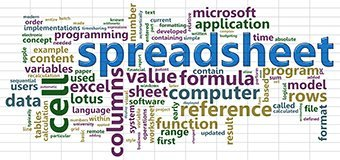Since my website does not allow non-US visitors,

I list my steps for reference for those who cannot download my macro-enabled demonstration workbook.

Step 1: Create a new workbook and save it as macro enabled.

Step 2: In the VB Editor, select the Sheet1 object and change the General object to the Worksheet object.

Step 3: Locate the Worksheet object’s SelectionChange event.

Step 4: Add the following code:

Private Sub Worksheet_SelectionChange(ByVal Target As Range)

If Not Intersect(Target, Range(“C1”)) Is Nothing Then
CallMacro NewName
End If
End Sub

Step 5: Insert a module

Step 6: Below the Option Explicit in your global declarations, add the following code:

Public Name As String
Public NewName As String
Dim r As Range

Step 7: Create the following UDF:

Public Function CallMacro(ByVal Name As String) As String

‘Ignore errors, in my code you will likely find a few
On Error Resume Next

‘The cells change event uses NewName
NewName = Name

Set r = Range(“C1”)
If Not IsError(Range(“C1”)) Then
If NewName = “ChangeA1” Then
Call ChangeA1
Else
Call ChangeB1
End If
End If
End Function

Step 8: Create the following procedure:

Public Sub ChangeA1()
Set r = Range(“C1”)
r.Offset(0, -2) = r.Offset(0, -2) + 1
r.Offset(0, -2).Interior.Color = 255
End Sub

Step 9: Copy the Step A code and modify bolded items

Public Sub ChangeB1()
Set r = Range(“C1”)
r.Offset(0, –1) = r.Offset(0, –1) + 1
r.Offset(0, –1).Interior.Color = 5287936
End Sub

Step 10: Change Excel’s Calculation Options. Select the File tab > Options command. In the Formulas category:
Enable Iterative Calculations
Increase the Maximum Iterations: 32,767
Maximum Change: 1
Close the options dialog.

Step 11: Save your workbook as macro enabled.

Step 12: Run/Test the macro
Choose the Debug menu > Compile command to run a syntax check.
In cell C1 create a formula that calls either ChangeA1 or ChangeB1. The syntax to the UDF is
=CallMacro(“ChangeA1”)
In cell D1 I type the number 2
In cell C1 the formula could read:
=IF(D1>1,CallMacro(“ChangeA1”),CallMacro(“ChangeB1”))

I encourage others to test my results and email me feedback.* Footnotes:

Chip Pearson on UDFs: http://www.cpearson.com/Excel/WritingFunctionsInVBA.aspx

“A UDF can only return a value to the cell(s) whence it was called — it must not modify the contents or formatting of any cell and must not modify the operating environment of Excel. If you attempt to change anything, the function will terminate immediately and return a #VALUE error to the calling cell”

Ozgrid on UDFs: https://www.ozgrid.com/VBA/Functions.htm#UDFIntro

“They cannot be used to try and change any part of another cell in any way at all. This means a UDF cannot place a value into any other cell except the cell housing the UDF”

Originally published by: Excel User Defined Function (UDF) Calls A Macro To Change A Value In Another Cell

# Excel BudgetsWhen speaking with Excel students, one of the most commonly asked Excel questions is: “How do I create a budget?”. My advice would be, if your boss asks you to create a department budget your first question should be “Okay, will the CFO provide a template for this project” (the answer should be yes). The second question might be (if there is no template), “can I base my forecast on a previous spreadsheet you provide”. However, assuming there is no template or previous spreadsheet, this tutorial will delve into the topic of basic financial modelling with Excel.

Budgeting is a broad topic and the approach taken will vary depending on the type of business. Budgeting for a manufacturer is not the same a budgeting for a non-profit organization. For simplicity this tutorial will focus on a Finance department in a small organization (20 employees) that sells a service. For readers who require a manufacturing budget or a marketing budget, please email me your requests and I will improve this tutorial in the next iteration.

## Components Of A Budget

A typical budget will likely include income and expenses. For simplicity, this example will ignore the income components and focus on modelling the expense items.

When constructing a budget for expenses we typically categorize departmental expenses in to two categories: operating expenses and non-operating expenses. Operating expenses typically fall into two categories: direct costs and indirect costs, which may be further classified as fixed or variable in reports.One of the most common direct fixed costs in your budget should include items like salaries and rent. Direct variable costs may include travel and mail. As much as your department has direct costs, they also account for indirect costs like electricity and selling, general, and administrative expenses. Though it is unlikely your boss requires this much detail, in this tutorial I also include a capital expenditures (CapEx) budget, which includes depreciation on computers and furniture.

Where a budget needs to show an annual number, this value is typically based on monthly details. Think of the annual budget as the end result of calculating each department’s monthly expenses. Your budget will likely be consolidated with other departments budgets and show up as line items on the income statement, balance sheet, and used in financial ratio analysis.

Though it’s possible to generate an entire budget using a single worksheet tab, best-practice would be to separate each line item to its own sheet tab and consolidate that data into the front summary budget sheet. The CFO may then take five departmental workbooks and consolidate those budgets into a ‘master’ workbook.

Suggestion: When viewing a spreadsheet you did not create, I recommend beginning your review by selecting the Formulas tab of the ribbon > Show formulas command. The keyboard shortcut to show formulas is hold the Ctrl key, tap and release the ~ (tilde key – upper left of keyboard). Ctrl~ a second time will hide the formulas and show the values.

The second worksheet in this example lists employees by job title, their annual salaries as a variable input, and formulas to show these salaries as monthly costs, with quarterly summaries as well. When modelling be aware that a well designed model should use color, borders, and bold fonts to improve readability. In this model blue numbers are variable inputs. Light grey cells are formulas based on the variable inputs. On the personnel sheet, several key cells use named ranges to simplify formulas on subsequent worksheet tabs. Best-practice would be to show a legend key that lists the named ranges. To improve readability of the quarterly values, consider using the group and outline feature to allow your boss to easily collapse monthly values to quarterly summaries.

In this tutorial the company is generous and pays 100% of a benefits plan, which should then be reflected in the budget. Cell A10 is a direct fixed expense value that feeds the calculation in cell S10. Notice in cell A10 I recommend a custom cell format to include the words Per Employee in the label.

Note that the end result of step 1 is the value on the Personnel sheet in cell S12, which feeds cell R2 on the Operating Expense Budget sheet tab.

### Step 2: Calculate the Operating Expenses

Where a general ledger would break operating expenses into monthly items, in this example I have annualized the data (for simplicity). The total annual operating expense items on the OpEx Details sheet tab produce a value that feeds the Operating Expense sheet tab cell R3. This example lists common operating expense accounts but your own list will be different. Also, you should ask if required to include Selling, General and Administrative allocations in your own budget.

As a final note, although Excel offers the ability to add comments to cells, notice on the OpEx Details tab and the CapEx Details sheet tab I have included a column labelled Notes. I believe that a Notes column adds clarity and improves readability compared to using Excel’s notes or comments feature. Notes should list any assumptions that are not obvious.

### Step 3: Calculate Rent Expenses

It is highly unlikely that your boss expects you to provide your rent expense in your budget. Rent is a part of operating expenses listed in the cash budget, which will impact the Cash line item on the balance sheet.
On the RentExpense sheet tab we lay out variable inputs to calculate the total rent allocation in cell Q9. To improve the readability of the formula in C9, the model uses named ranges in column B.

The cost per square foot will likely be influenced by at least two factors: 1) The building’s street address; 2) The class of office (A, B, C, etc). In this example I show an average price for a class A office in midtown NYC.
Disclaimer: I am not a Certified Public Account. In my opinion, if your company owns the building and does not pay rent, then consider using the property tax plus property insurance plus maintenance costs plus building salaries here to approximate a value for the cost per square foot.

### Step 4: Calculate Depreciation

It is highly unlikely that your boss expects you to forecast your department’s depreciation expense allocations. Depreciation is a non-cash operating expense will affect the Asset line on the balance sheet.
To calculate depreciation, we need a list of capital expenditures, which are items that have a life longer than one year and are amortized according to IRS guidelines. CapEx items often include computer systems, telephone systems, copiers, and furniture. In the Excel Class budget the CapEx details sheet tab lists these one time expenses and assumptions.

To obtain the annual value, the Capital Expenses sheet tab lays out the line items as Computer, Furniture and Miscellaneous, given that some items have different depreciation requirements.

Of course, this model still has room for improvement. SavvyExcel developers will create a separate worksheet tab labelled ‘Assumptions’. In this model there are many formulas where I have hard coded a 20% allocation for shared resources. If that allocation were to change, I would then need to update many formulas. Were I to reference a value in a cell on an allocation assumptions worksheet then I would only have to change the value in a single location. This model does not include a seasonality factor. If I had included an assumptions worksheet tab, for some businesses I would include a seasonality multiplier. A seasonality multiplier would allow us to increase or decrease some variable operating expenses depending on the month.

I hope that some of you will find this budgeting exercise helpful. Thank you for your time! If you find any errors or omissions in this Excel budget example please contact me with proposed corrections or suggestions.

Originally posted: https://excelclasstraining.com/excel-budgets/

# Two Criteria Lookups

In the process of teaching Excel over the past 20 years I have found that most students are often shocked and unaware that Vlookup and Index/Match can only locate the first item in a list. They find this unacceptable and I would tend to agree. When a value repeats in many rows, Microsoft suggest you use the upcoming Xlookup function to locate what is known as the ‘nth’ occurrence, but most Office365 installations will not offer that feature before July 2020 – at the earliest! For a long time there has been a workaround that does not require Xlookup, which despite Microsoft’s best intentions likely is not necessary!

In the “real world” a list typically includes items that repeat. Consider an invoice, it will typically include many line items for a single invoice number. Where one column may contain a repeating invoice number, the line items in rows could be for the same product with different size or color options. I recently came across several clients who require this functionality. While the topic of this blog post is beyond most students needs, I include it here because the class notes have finite space. This post assumes that students have taken the advanced Excel class and already understand how to work with Index and Match functions.

To follow along with the example, I recommend you download this sample workbook: Index Match Two Criteria

In this example notice that column A contains invoice numbers that repeat (orange cells – first criteria), and column B shows a unique price (second criteria). The goal is to locate the correct quantity for each invoiced item based on its price.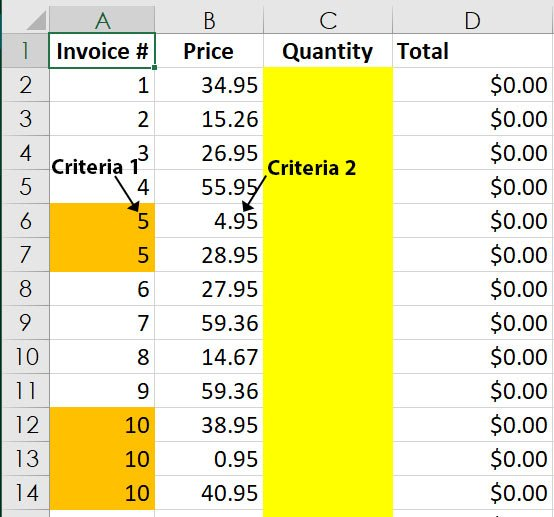The formula required in cell C2 is:

=INDEX(DataToSearch!\$F\$1:\$F\$5061,MATCH(1,(InvoiceTwoCriteria!A2=DataToSearch!\$B\$1:\$B\$5061)*(InvoiceTwoCriteria!B2=DataToSearch!\$E\$1:\$E\$5061),0))

Given the formula’s complexity, I also recommend simplifying the readability by using named ranges, which could read:

=INDEX(Quantity,MATCH(1,(InvoiceTwoCriteria!A2=InvoiceNumber)*(InvoiceTwoCriteria!B2=Price),0))

After copying one of these formulas, take a moment to study and read it but… do not simply press the enter key, this formula requires the use of an array – hold both Ctrl+Shift while pressing the Enter key. As you study the format the formula, pay special attention to the placement of the open and close parentheses!

Because it is any array formula, we cannot reference an entire column, it requires a fixed range with absolute references (recall that named ranges are also absolute references). Also bear in mind that array formulas have limitations that can be found at this URL: https://docs.microsoft.com/en-us/office/troubleshoot/excel/arrays-limitations

The way to read and interpret the formula is:

Index (return) a value from column F. The Match lookup value of 1 creates a matrix of 1/0 values. The Match lookup array for criteria one reads: Look for the value in cell A2 (invoice #1) by searching cells B1 to B5061. The multiplication symbol before the second lookup array is treated like an AND operator. The second lookup array reads: find the price in cell B2 (34.95) by searching cells E1 to E5061. When the matrix returns a 1 match (true) for the invoice in column B, and a 1 match (true) for the price in column e, then return the corresponding value from column F (price). And if some of you are wondering, yes, this can be extended to include three criteria if needed!

If you would like to practice creating these types of formulas, download the following workbook and enable macros. Note that the submit answer feature is not expecting you use named ranges, though not incorrect the program is looking for range references, not named ranges! Index Match Two Criteria Lab

This post was originally published here: https://excelclasstraining.com/two-criteria-lookups/

Originally published here

# SumProduct Function Applications

When teaching Excel classes students occasionally ask about the SumProduct function. There are many aspects of SumProduct that mirror the SumIfs functions capabilities but SumProduct can produce different answers when combined with the unary operator. This tutorial is not meant to be a thorough discussion of SumProduct but is meant to provide an addendum to class notes.

I have provided a picture of the worksheet for those who cannot open Excel workbooks:### SumProduct – Example 1

The SumProduct function multiplies one array by another array. An array is typically a range of cells in a single column. In the first example imagine that our goal is to calculate the total gross pay for all employees in all divisions and departments. The ‘old fashioned’ Excel approach is to write a formula to calculate hours * rate for each employee (cells G2:G21) then Sum those values. In this Level 1 example, we would need to generate 20 formulas in 20 cells to find the answer, where a SumProduct function calculates the result with less effort.

With SumProduct
=SUMPRODUCT(E:E,F:F)

Without SumProduct
=(E2*F2)+(E3*F3)+(E4*F4)+(E5*F5)+(E6*F6)+(E7*F7)+(E8*F8)+(E9*F9)+(E10*F10)+(E11*F11)+(E12*F12)+(E13*F13)+(E14*F14)+(E15*F15)+(E16*F16)+(E17*F17)+(E18*F18)+(E19*F19)+(E20*F20)+(E21*F21)

### SumProduct – Example 2

About fifteen years ago Excel did not offer a SumIfs function and SumProduct was an alternative to using an array formula. Like the SumIfs function, SumProduct can work conditionally using criteria. Where SumIfs only allows AND criteria, SumProduct also supports OR criteria (but this generally double counts and generates incorrect results). Several studies have shown that SumIfs functions calculate significantly faster than SumProduct functions.

In example 2 imagine that we want to only sum the gross pay if the division equals Maine and the department equals Sales. In this example the asterisk (*) between Array1 and Array2 operates as an AND operator. The formula reads, If column C contains “Maine” AND Column D contains “Sales”, then Sum the value in column E times column F. If we use a plus (+) instead of an asterisk, this represents an OR criteria but double counts the products of Maine and Sales.

With SumProduct
=SUMPRODUCT((C:C=”Maine”)*(D:D=”Sales”),E:E,F:F)

With SumIfs
=SUMIFS(G:G,C:C,”Maine”,D:D,”Sales”)

Array Formula Sum If
{=SUM(IF((C:C=”Maine”)*(D:D=”Sales”),E:E*F:F))}

### SumProduct – Example 3

At times we see a double hyphen (- -) in a formula. In Excel the double hyphen is known as a unary operator. The purpose of the unary operator is to force text values to 0/1 values where zero means false and one means true.

SumProduct Formula
=SUMPRODUCT(–(C:C=”Maine”)*–(D:D=”Sales”),E:E,F:F)

Like example 2 except now the – operator converts values where a cell contains Maine or Sales to 0 for false and 1 for true, then uses the corresponding 1 values to determine which values in columns E and F are multiplied and summed.

SumProduct is also used to compare values in two columns to count how many words or numbers match (typically columns in separate workbooks). In general, do not sort the data, this technique compares corresponding values within the same row.

SumProduct Formula
=SUMPRODUCT(–(\$C:\$C=”Maine”),–(\$I:\$I=”Maine”))

This formula asks Excel to compare the values in column C to the values in column I for the word Maine. The unary operator converts results to 0 for false or 1 for true, and then the SumProduct function sums the values for 1/true.

SumProduct can also compare the value in two columns to count identical values

SumProduct Formula
=SUMPRODUCT(–(E:E=40),–(K:K=40))

Like the previous example, this formula asks SumProduct to sum the 1 values if the numbers in column E and K are identical and equal 40.

### SumProduct – Example 4

The most valuable use of the SumProduct is to calculate a weighted average (this question only occurs about once every ten years). The straight average sums the values and divides by the count. A weighted average considers some values have a higher weight. In this example imagine the weighted average is based on the count of Divisions, where there are six Connecticut values, four Maine values, and five New Hampshire and five Vermont values.

In this example the SumProduct weights the average gross pay based on the count of divisions.

SumProduct Formula – Longhand notation for weighted average
=SUMPRODUCT({780,780,860,504,589,504,350,213,860,589,472.15,176,860,880,443.75,880,472.15,780,840,350},{4,6,4,6,5,6,5,6,5,6,5,5,5,5,5,5,6,4,4,5})/SUM(H2:H21)

SumProduct Formula – Shorthand notation for weighted average
=SUMPRODUCT(E2:E21,F2:F21,H2:H21)/SUM(H2:H21)

This tutorial was originally posted here: https://excelclasstraining.com/data-tables-and-interactive-charts/

The Excel Class blog provides an opportunity to supplement the material we teach in our class. This tutorial assumes our readers are familiar with named ranges. (See: Advanced Excel Class)

Excel’s data table feature is a what-if analysis tool that allows users to create tables (rows/columns) of results based on a one or two variable formula. Data tables are useful for simple calculations like future value tables or mortgage payment tables to examine different scenarios based on varying input assumptions. Our previous post examined array formulas with vlookup (Vlookup Array Formula Tutorial) and data tables are an extension of the discussion of array formulas.

In the following example we use a data table to show rates of return for an investment and then use the data table as the basis of an interactive chart using an offset function in an array formula.

The formula for Future Value is:

FV = PV * (1 + i) ^ t

Where:

FV means future value

PV means present value

i is the interest rate

t is time

## To Create A Data Table

1. Set up variable inputs, optionally (recommended) name the cells to use in the formula;2. To the right of the PV label, in cell B1, type a number like 1. In this example 1 means \$1, our present value.

3. In a separate cell, create the formula that references the named ranges. In this example the formula appears in cell C4 which will become the upper left corner of the data table;4. To the right and below the formula, add variable inputs;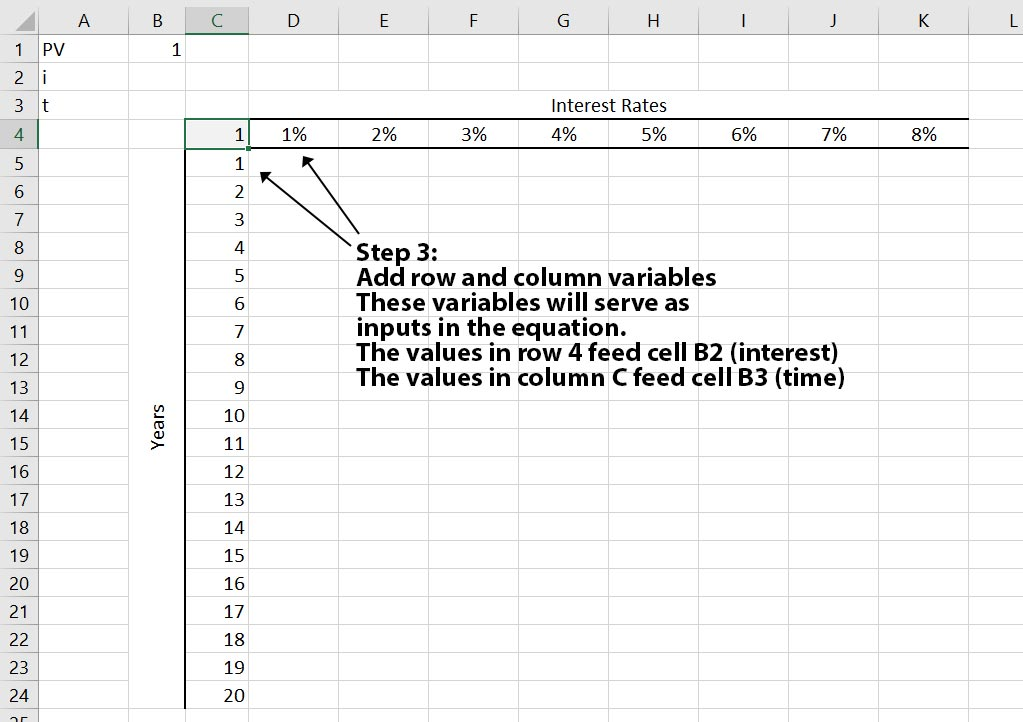5. Select the range to use for the data table, including the formula in the upper left corner;
In this example select cells C4:K24;

6. On the ribbon, select the Data tab > What If Analysis > Data Table command;
Select a row input cell (refer to a cell with a name); In this example, cell B2 is the row input (interest).
Select a column input cell (refer to a cell with a name); In this example, cell B3 is the column input (time).
Click the OK button to generate the data table.Note: The formulas produced by the data table in cells D5:K24 are array formulas. Be aware that array formulas remove the ability to insert or delete a row or a column inside the data table.

## To Create An Interactive Chart Based On The Data Table

1. In cell C26, type a 0 (zero);

2. Select (highlight) cells D26:K26;

3. Type an = (equals) sign;

Enter the following formula:
=OFFSET(D5:K5,C26,0)

To create an array formula, hold the Ctrl+Shift keys and press the Enter key.

The offset function offsets the range D5:K5 by the value in cell C26 (zero rows)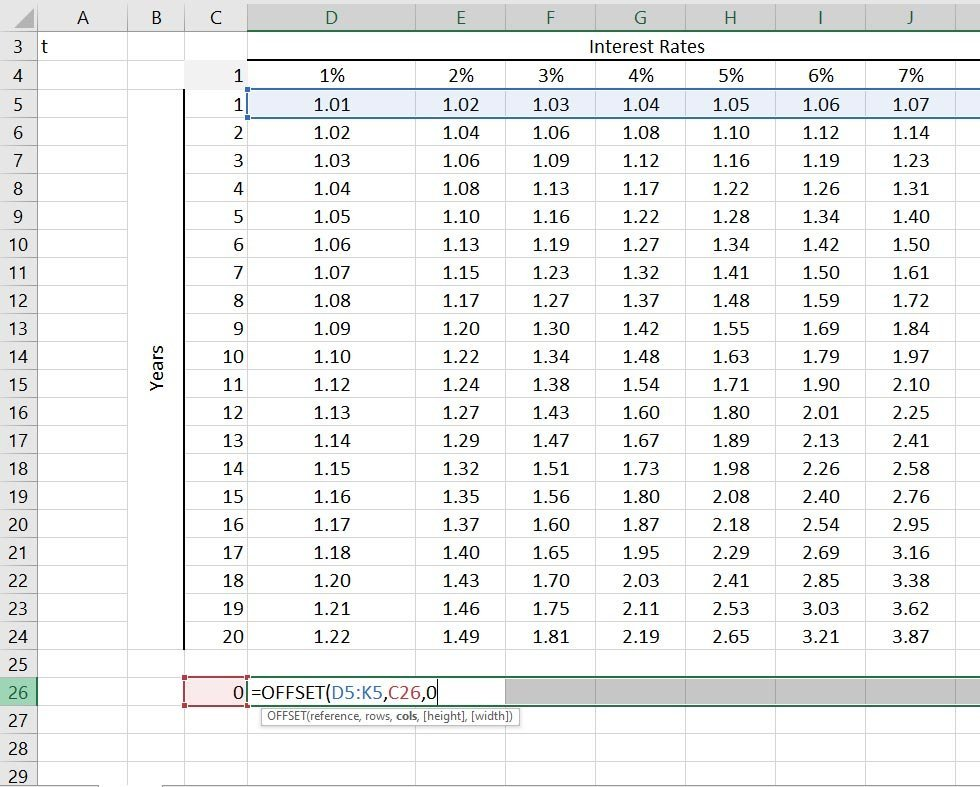4. To add an interactive spinner control, show the Developer tab of the ribbon. To show the Developer tab, right click any tab of the ribbon, choose the Customize Ribbon… command. In the Customize Excel Options dialog, check the Developer tab.

5. On the Developer tab of the ribbon, select the Insert > Form Controls > Spinner command.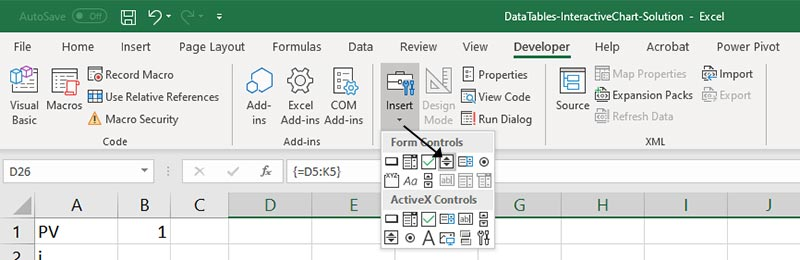6. Click and drag a small spinner control in a cell to the left of cell C26.

7. Right click the spinner control, left click the Format Control command.

In the Format Control dialog:

Set the minimum value to 0

Set the maximum value to 20 (the number of years in column C)

Set the link cell to cell C26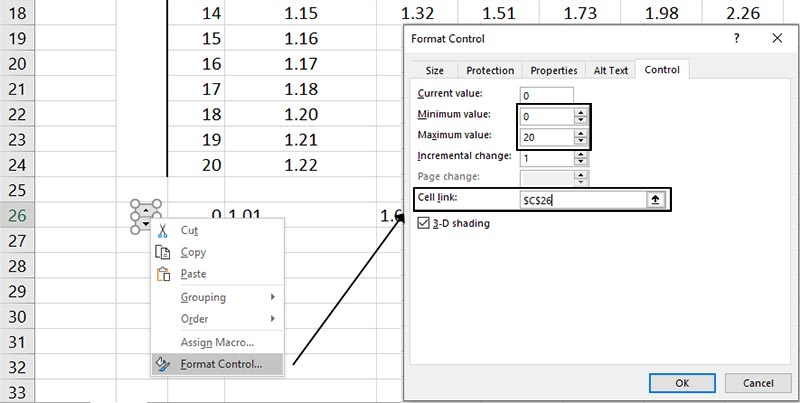8. The final step is to create the interactive chart.

Select cells D26:K26

On the ribbon, select the Insert tab > 2D Line Chart command9. To see the chart become interactive, click the up arrow on the spinner control to increase the value in cell C26.

Note that as the value increases the offset function plots different rows of the data table in the chart.

Final note: I am obligated to give credit to Tushar Mehta for his excellent charting tutorials. Learn more about Tushar’s chart tutorials here: http://tushar-mehta.com/excel/charts/ In fact, I always encourage Excel students to study Tushar’s tutorials on: 3D Surface Charts, Normal Distribution (Bell Curves), Waterfall (Cash flows), and Values That Differ By Magnitude.

In the process of teaching Excel classes, many students ask:

How can I write a single vlookup function that returns multiple values to the right, instead of a single value, which causes me to write many vlookup functions in many columns.

This tutorial does not teach vlookup, that feature is taught in the Introduction and Advanced classes. Here we focus on vlookup and array formulas. The purpose of this tutorial is to supplement the class notes.

When performing calculations in Excel, typically a single cell contains a formula with functions that return a single value. An array formula is different in that it will typically include many cells (rows or columns) and return many values. Where I would teach students to press the enter key to commit an edit, to create an array formula we are required to hold Ctrl+Shift+Enter.

Vlookup Array Workbook

Though not required, I suggest when you open this workbook, click the Enable macros command which will create two windows and tile them side by side.
In this example, the idea is to write a single vlookup function on the VlookupOrders sheet. The single vlookup will find the order number on the Data sheet and return three values, the Company Name, the Country and the Sale.

## Example Of Data In Vlookup Array Workbook1) On the VlookupOrders worksheet tab, select/highlight cells C2:E2
2) Create the following formula:
=VLOOKUP(B2,Data!B:F,{3,2,5},0)Note: For the third argument, the col_index_num, we are allowed to use as many values as needed. In this example I am trying to illustrate that as much as I can return three columns to three cells — in any sequence — we can choose any number of columns as needed. However, to vlookup four columns, in step 1, select/highlight four cells. Also note that we cannot simply add parentheses around the values of the col_index_num, use curly braces {} (squiggly brackets – typically found to the right of the letter P on your keyboard).

3) To convert this to an array formula, hold the Ctrl+Shift keys and press the Enter key. The array formula adds {} (curly braces) around the vlookup formula.

A final word of warning: Array formulas have drawbacks. One drawback is that array formulas recalculate a bit slowly over thousands of rows. A bigger drawback is that we have lost the ability to insert a new column within the arrayed formula range. To verify the loss of the ability to insert a column inside the array, on the VlookupOrders sheet, right click column D and left click the Insert command.

Excel Class receives requests for Microsoft Excel VBA training courses from every corner of the country. We are pleased to announce that our new exclusive series: The Excel VBA webinar is now available online.

Our training sessions begin once a month at different start times. Each session lasts two hours and includes no less than 30 minutes of homework to practice between sessions. In total the classes run for 12 weeks. Since we realize you may not be able to attend 12 consecutive weeks we allow you to retake the class an unlimited number of times and you can start in one class and skip into others classes (by providing us with advance notice). We offer exclusive video libraries where students can log in to a secured area to review videos of your class and download example workbooks.

Though our New York class runs for 28 hours, our webinar is spread across 48 hours to allow more time to review homework, provide time for questions and answers, and to go into further detail.

VBA Training Online Press Release

Originally seen here

Microsoft Excel works similarly to using a calculator but there are important differences. On a calculator to perform a calculation you might type numbers, then math symbols like plus (+) or minus (-) and then you would press the equal key to complete your calculation.

In Excel we don’t end with the equal sign, we begin with the equal sign. Additionally, you need to be aware of the mathematical order of operations, commonly associated with the acronym PEMDAS (Please Excuse My Dear Aunt Sally).

What the order of operations theorem states is that parenthesis () overrides exponentiation (^) which has a higher precedence than multiplication (*) or division (/) and that multiplication and division have a higher order of precedence than addition (+) and subtraction (-). For those of us who don’t have degrees in rocket science, exponentiation is the term used to say raise one number to the power of another number, like 28 which would equal 256 (2*2 eight times).

To see how PEMDAS applies to real world examples let’s look at two formulas:

Example of PEMDAS:

= 2 + 3 * 4

Most people would read that formula from left to right and calculate 2+3 equals 5 and 5 times 4 equals 20, and it wouldn’t be entirely wrong. However, because multiplication has a higher order of precedence than addition, Excel will first calculate 3*4 equals 12 and 12+2 equals 14.

Therefore, if you want the formula to produce 20 instead of 14 you need to override the order of operations by grouping the 2+3 terms inside parentheses as in:

= (2 + 3) * 4

In Excel formulas it is common to see many many parentheses. That is because most functions (predefined formulas) use parentheses to enclose the arguments (inputs) and those arguments may nest other functions in parentheses and each function may require parenthesis around the inputs. For example,

=IF(D2=”R”,INDEX(\$K\$3:\$K\$8,MATCH(B2,\$J\$3:\$J\$8,FALSE)*C2),IF(D2=”C”,INDEX(\$H\$3:\$H\$8,MATCH(B2,\$G\$3:\$G\$8,FALSE)*C2),IF(D2=”H”,INDEX(\$N\$3:\$N\$8,MATCH(B2,\$M\$3:\$M\$8,FALSE)*C2))))

What is important to realize about using parentheses is that if you use () symbols you have to be careful to balance every open parentheses with a close parenthesis or the formula will produce an error. If you’re unsure of why this formula uses \$ signs in the cell references, please refer back to our blog about absolute versus relative cell references.